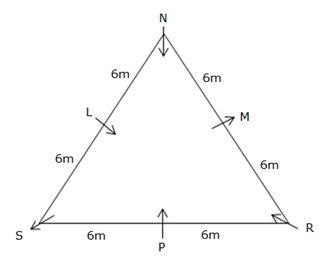# SBI PO Prelims Reasoning Questions 2019 (Day-40)

Dear Aspirants, Our IBPS Guide team is providing new series of Reasoning Questions for SBI PO 2019 so the aspirants can practice it on a daily basis. These questions are framed by our skilled experts after understanding your needs thoroughly. Aspirants can practice these new series questions daily to familiarize with the exact exam pattern and make your preparation effective.

[WpProQuiz 6256]

### Click Here for SBI PO Pre 2019 High-Quality Mocks Exactly on SBI Standard

Directions (1-5): Study the following arrangement carefully and answer the questions given below.

F # O U 7 D \$ V 8 C % B < K 1 S 6 £ Q 2 R C M 4 Y A & E Z 9 H I W 5 *

1) Which of the following is exactly in the middle between first vowel in this series from the left end and Q in the above arrangement?

a) C

b) %

c) 8

d) 1

e) None of these

2) Which of the following element will be seventh to the left of twenty third element from the left end?

a) 6

b) K

c) 1

d) C

e) S

3) Which of the following element will be exactly in the middle of the sequence?

a) £

b) 6

c) Q

d) None

e) S

4) How many such symbols are there in the above arrangement, each of which is immediately followed by a vowel but not preceded by a vowel?

a) Three

b) Two

c) None

d) More than three

e) One

5) If the above sequence is written in reverse order then which of the following will be sixth to the right of sixteenth element from the right end?

a) C

b) K

c) A

d) C

e) None of these

Directions (6-10): Study the following arrangement carefully and answer the questions given below.

Six persons – L, M, N, P, R and S are sitting in an equilateral triangular table. Three of them sit at the corner of the triangular table and three of them sit at the middle of the side. Some of them are facing the centre and some of them are facing outward of the table. Not more than two people sitting together face same direction. The perimeter of the triangle is 36m.

Note: Perimeter of an equilateral triangle = 3a, where ‘a’ is the side of a triangle.

N is sits in a corner and faces towards the centre. Distance between P and R is 6m, where both of them are not immediate neighbours of N. Only one person sits between R and N. S sits second to the left of R. If S walks for a distance of 12m towards its right it will end up with N. L faces inside. M who is an immediate neighbour of R sits second to the right of P.

6) What is the shortest distance between N and P?

a) √108 m

b) 5√6 m

c) 6√3 m

d) Both (a) and (b)

e) Both (a) and (c)

7) Who among the following sits second to the right of N?

a) R

b) S

c) P

d) Cannot be determined

e) None of these

8) If the distance between M and R is changed to 8m then what will be the distance between S and N by satisfying the condition of equilateral triangle?

a) 4m

b) 8m

c) 16m

d) √180m

e) Cannot be determined

9) Who among the following sits to the immediate right of M?

a) N

b) R

c) L

d) S

e) Cannot be determined

10) Four of the following are alike in a certain way. Who among the following does not belong to the group?

a) P

b) R

c) N

d) M

e) L

Directions (1-5):

F # O U 7 D \$ V 8 C %B < K 1 S 6 £ Q 2 R C M 4 Y A & E Z 9 H I W 5 *

F # O U 7 D \$ V 8 C % B < K 1 S 6 £ Q 2 R C M 4 Y A & E Z 9 H I W 5 *

F # O U 7 D \$ V 8 C % B < K 1 S 6 £ Q 2 R C M 4 Y A & E Z 9 H I W 5 *

F # O U 7 D \$ V 8 C % B < K 1 S 6 £ Q 2 R C M 4 Y A & E Z 9 H I W 5 *

* 5 W I H 9 Z E & A Y 4 M C R 2 Q £ 6 S 1 K < B % C 8 V \$ D 7 U O # F

Directions (6-10):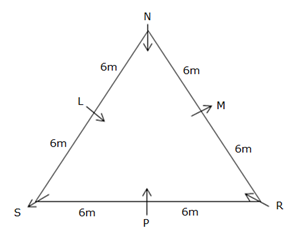N is sits in a corner and faces towards the centre. Distance between P and R is 6m, where both of them are not immediate neighbours of N. Only one person sits between R and N.

Here we get two cases Case (1) and Case (2).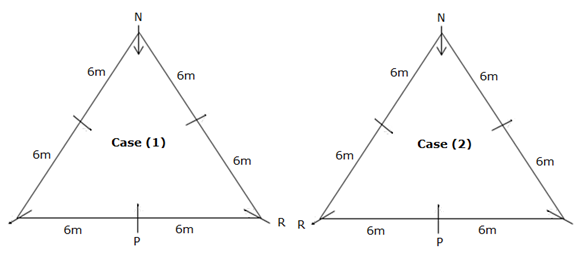S sits second to the left of R. M is an immediate neighbour of R. If S walks for a distance of 12m along the perimeter of the triangle towards its right it will end up with N.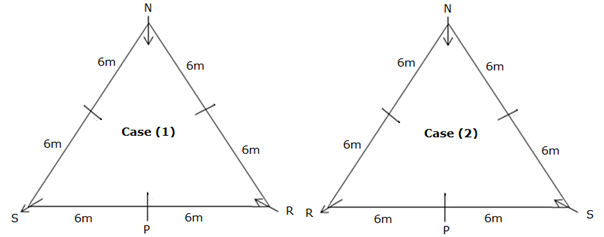M who is an immediate neighbour of R sits second to the right of P.L faces inside.

Here, Case (2) gets eliminated as no three persons sitting together can face same direction.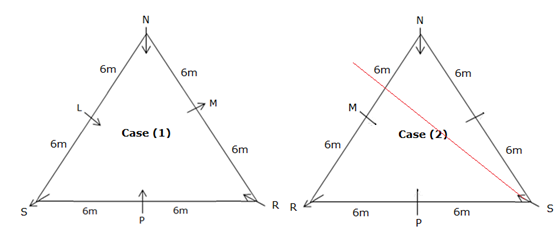And the final arrangement is,